# Texas Go Math Grade 4 Lesson 5.4 Answer Key Use Benchmarks to Determine Reasonableness

Refer to our Texas Go Math Grade 4 Answer Key Pdf to score good marks in the exams. Test yourself by practicing the problems from Texas Go Math Grade 4 Lesson 5.4 Answer Key Use Benchmarks to Determine Reasonableness.

## Texas Go Math Grade 4 Lesson 5.4 Answer Key Use Benchmarks to Determine Reasonableness

Essential Question

How can you find and record sums and differences of fractions?

To add fractions there are Three Simple Steps:
1. Step 1: Make sure the bottom numbers (the denominators) are the same.
2. Step 2: Add the top numbers (the numerators), put that answer over the denominator.
3. Step 3: Simplify the fraction (if possible)
There are 3 simple steps to subtract fractions
1. Make sure the bottom numbers (the denominators) are the same.
2. Subtract the top numbers (the numerators). Put the answer over the same denominator.
3. Simplify the fraction (if needed).

Unlock the Problem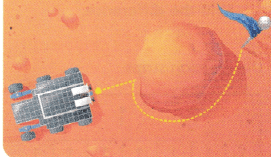A rover considers many possible paths before choosing the safest path toward its goal. A rover moved yard in a straight line, and then yard around a rock to reach its goal. How far did it travel?

Find the sum.

MODEL IT

Use fraction strips.

Think: The rover moved 2 sixth yard and then 5 sixth yard. Shade 2 sixth-size pieces and then 5 sixth-size pieces.So, the rover traveled _________ yards to reach its goal.
So, the rover traveled $$\frac{7}{6}$$  yards to reach its goal.$$\frac{2}{6}$$ + $$\frac{5}{6}$$  = $$\frac{7}{6}$$
So, the rover traveled $$\frac{7}{6}$$ yards to reach its goal.

RECORD IT

Write the sum.
_________ + _________ = $$\frac{7}{6}$$
Rename $$\frac{7}{6}$$ as a mixed number.
Think: The model shows 1 whole yard and 1 sixth yard.
$$\frac{7}{6}$$ = ___________
$$\frac{2}{6}$$ + $$\frac{5}{6}$$  = $$\frac{7}{6}$$
Rename $$\frac{7}{6}$$ as a mixed number.
Think: The model shows 1 whole yard and 1 sixth yard.
$$\frac{7}{6}$$ = 1$$\frac{1}{6}$$

Math Talk

Mathematical Processes
Explain how you know $$\frac{5}{6}$$ is greater than $$\frac{1}{2}$$.

Determine whether the sum is reasonable.

Compare the addends to the benchmarks 0, $$\frac{1}{2}$$, and 1.$$\frac{2}{6}$$ is greater than $$\frac{1}{2}$$. and less than $$\frac{1}{2}$$
The sum is greater than 0 + $$\frac{1}{2}$$ = $$\frac{1}{2}$$ .
The sum is less than $$\frac{1}{2}$$ + 1 = $$\frac{3}{2}$$ .
So, 1$$\frac{1}{6}$$ is a reasonable sum.$$\frac{5}{6}$$ is Greater than $$\frac{1}{2}$$ and lesser than 1.

Example

A rover must move $$\frac{5}{8}$$ mile to reach its goal. The rover moves $$\frac{1}{8}$$ mile toward its goal. How much farther must the rover move to reach its goal?$$\frac{5}{8}$$ – $$\frac{1}{8}$$ = $$\frac{4}{8}$$

(A) Find the difference.
$$\frac{5}{8}$$ – $$\frac{1}{8}$$ = $$\frac{4}{8}$$
$$\frac{4}{8}$$ farther must the rover move to reach its goal

MODEL IT

Use fraction strips.So, the rover must ___________ move mile farther.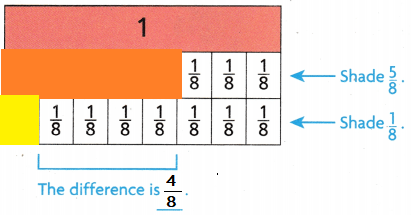So, the rover must $$\frac{4}{8}$$ move mile farther.

RECORD IT

Write the difference.
__________ – ____________ = $$\frac{4}{8}$$
$$\frac{5}{8}$$ – $$\frac{1}{8}$$ = $$\frac{4}{8}$$

(B) Determine whether the difference is reasonable.

Compare the fractions to the benchmarks 0, $$\frac{1}{4}$$, $$\frac{3}{4}$$, and 1.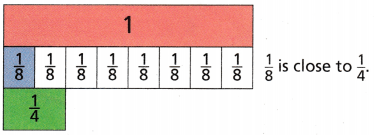$$\frac{1}{8}$$ is greater than 0 and less than $$\frac{1}{4}$$.
The difference is greater than 0 + $$\frac{1}{4}$$ = __________.
The difference is less than $$\frac{1}{4}+\frac{3}{4}$$ = ____________.
So, $$\frac{4}{8}$$ is a reasonable difference.
$$\frac{1}{8}$$ is greater than 0 and less than $$\frac{1}{4}$$.
The difference is greater than 0 + $$\frac{1}{4}$$ = $$\frac{1}{4}$$ .
The difference is less than $$\frac{1}{4}+\frac{3}{4}$$ = 1.
So, $$\frac{4}{8}$$ is a reasonable difference.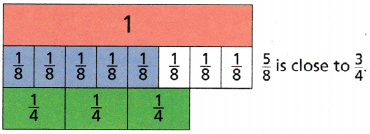$$\frac{5}{8}$$ is ____________ than $$\frac{1}{4}$$ and __________ than $$\frac{3}{4}$$.
$$\frac{5}{8}$$ is Greater than $$\frac{1}{4}$$ and lesser than $$\frac{3}{4}$$.

Share and Show

A rover needs to move $$\frac{9}{10}$$ mile to a crater. It moves $$\frac{4}{10}$$ mile ‘toward the crater. How much farther does it need to move to reach the crater?

• Model the difference.
• Write the difference.

$$\frac{9}{10}-\frac{4}{10}$$ = ____________$$\frac{9}{10}-\frac{4}{10}$$ = $$\frac{5}{10}$$

Question 2.
$$\frac{5}{12}+\frac{4}{12}$$ = __________
$$\frac{5}{12}+\frac{4}{12}$$ = $$\frac{9}{12}$$
Explanation:
It is reasonable because $$\frac{9}{12}$$ is lesser than 1 and greater than 0

Question 3.
$$\frac{4}{6}-\frac{2}{6}$$ = __________
$$\frac{4}{6}-\frac{2}{6}$$ = $$\frac{2}{6}$$
Explanation:
It is reasonable because $$\frac{2}{6}$$ is lesser than 1 and greater than 0

Question 4.
$$\frac{3}{8}+\frac{7}{8}$$ = __________
$$\frac{3}{8}+\frac{7}{8}$$ = $$\frac{9}{8}$$
Explanation:
It is not reasonable because $$\frac{9}{8}$$ is Greater than 1 and greater than 0

Unlock the Problem

H.O.T. Apply Multi-Step in our solar system, $$\frac{2}{8}$$ of the planets have no moons, $$\frac{1}{8}$$ have 1 moon, $$\frac{1}{8}$$ have 2 moons, and $$\frac{1}{8}$$ have 13 moons. What fraction of the planets have 0, 1, 2, or 13 moons?
(A) $$\frac{5}{8}$$
(B) $$\frac{4}{8}$$
(C) $$\frac{3}{8}$$
(D) $$\frac{2}{8}$$
Explanation:
$$\frac{2}{8}$$ + $$\frac{1}{8}$$ + $$\frac{1}{8}$$ + $$\frac{1}{8}$$ = $$\frac{5}{8}$$
$$\frac{5}{8}$$ fraction of the planets have 0, 1, 2, or 13 moons

a. What do you need to know?
fraction of the planets that have 0, 1, 2, or 13 moons.

b. What information are you given?
In our solar system, $$\frac{2}{8}$$ of the planets have no moons, $$\frac{1}{8}$$ have 1 moon, $$\frac{1}{8}$$ have 2 moons, and $$\frac{1}{8}$$ have 13 moons. Is the information given

c. Write the addition problem you will use to solve this problem.
$$\frac{2}{8}$$ + $$\frac{1}{8}$$ + $$\frac{1}{8}$$ + $$\frac{1}{8}$$ = $$\frac{5}{8}$$e. Fill in the bubble for the correct answer choice above.

Question 6.
A man times the movement of a banana slug. It moves $$\frac{2}{6}$$ foot during the first minute. It then moves $$\frac{3}{6}$$ foot during the second minute. How far does the banana slug move in all?
(A) $$\frac{5}{12}$$ foot
(B) $$\frac{1}{6}$$ foot
(C) $$\frac{1}{12}$$ foot
(D) $$\frac{5}{6}$$ foot
Explanation:
$$\frac{2}{6}$$ + $$\frac{3}{6}$$ = $$\frac{5}{6}$$ foot
$$\frac{5}{6}$$ foot far does the banana slug move in all

Question 7.
One day $$\frac{3}{8}$$ of the students in Jack’s class ate toast for breakfast. Another $$\frac{1}{8}$$ of the students ate oatmeal. Jack added the fractions and found the sum was $$\frac{7}{8}$$. Which statement best describes the sum $$\frac{7}{8}$$?
(A) It is reasonable because $$\frac{1}{2}$$ + 0 = $$\frac{1}{2}$$.
(B) It is reasonable because $$\frac{1}{2}$$ + $$\frac{1}{2}$$ = 1.
(C) It is not reasonable because $$\frac{1}{2}$$ + 0 = $$\frac{1}{2}$$.
(D) It is not reasonable because $$\frac{1}{2}$$ + $$\frac{1}{2}$$ = 1.
Explanation:
$$\frac{3}{8}$$ + $$\frac{3}{8}$$ = $$\frac{4}{8}$$ = $$\frac{1}{2}$$.
It is not reasonable because $$\frac{1}{2}$$ + 0 = $$\frac{1}{2}$$.

Multi-Step Ms. Ryan buys $$\frac{7}{8}$$ yard of striped cloth. She uses $$\frac{3}{8}$$ yard to make a bag. Then she uses $$\frac{1}{8}$$ yard to make a belt. how much cloth does Ms. Ryan have left to make a hat?
(A) $$\frac{2}{8}$$ yard
(B) $$\frac{4}{8}$$ yard
(C) $$\frac{3}{8}$$ yard
(D) $$\frac{6}{8}$$ yard
Explanation:
$$\frac{3}{8}$$ + $$\frac{1}{8}$$  = $$\frac{4}{8}$$
$$\frac{7}{8}$$ – $$\frac{4}{8}$$ = $$\frac{3}{8}$$ yard
$$\frac{3}{8}$$ yard cloth does Ms. Ryan have left to make a hat

TEXAS Test Prep

Question 9.
Suppose a rover on Mars moved $$\frac{2}{6}$$ yard in a straight line. Then it moved $$\frac{5}{6}$$ yard around a rock, flow many more yards did the rover move around the rock than it moved in a straight line?
(A) $$\frac{3}{12}$$ yard
(B) $$\frac{3}{6}$$ yard
(C) $$\frac{7}{12}$$ yard
(D) 1$$\frac{1}{6}$$ yard
Explanation:
$$\frac{5}{6}$$ – $$\frac{2}{6}$$  = $$\frac{3}{6}$$ yard
$$\frac{3}{6}$$  more yards that the rover move around the rock than it moved in a straight line

### Texas Go Math Grade 4 Lesson 5.4 Homework and Practice Answer Key

Question 1.
Melina wants to finish $$\frac{6}{10}$$ of her math homework problems before dinner. She finishes $$\frac{4}{10}$$ of them. What fraction of her math problems does she still need to complete before dinner?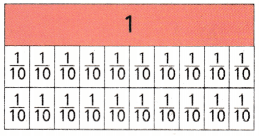• Model the difference.
• Write the difference.

$$\frac{6}{10}-\frac{4}{10}$$ = ___________$$\frac{6}{10}-\frac{4}{10}$$ = $$\frac{2}{10}$$

Question 2.
$$\frac{1}{6}+\frac{4}{6}$$ = ___________
$$\frac{1}{6}+\frac{4}{6}$$ = $$\frac{5}{6}$$
Explanation:
It is reasonable because $$\frac{5}{6}$$ is lesser than 1 and greater than 0

Question 3.
$$\frac{3}{4}-\frac{1}{4}$$ = ___________
$$\frac{3}{4}-\frac{1}{4}$$ = $$\frac{2}{4}$$
Explanation:
It is reasonable because $$\frac{2}{4}$$ is lesser than 1 and greater than 0

$$\frac{9}{12}-\frac{3}{12}$$ = ___________
$$\frac{9}{12}-\frac{3}{12}$$ = $$\frac{6}{12}$$
Explanation:
It is reasonable because $$\frac{6}{12}$$ is lesser than 1 and greater than 0

Question 5.
$$\frac{3}{6}+\frac{2}{6}$$ = ___________
$$\frac{3}{6}+\frac{2}{6}$$ = $$\frac{5}{6}$$
Explanation:
It is reasonable because $$\frac{5}{6}$$ is lesser than 1 and greater than 0

Problem Solving

Question 6.
In Joe’s family, $$\frac{2}{6}$$ of the people have blue eves and $$\frac{3}{6}$$ of the people have brown eyes. What fraction of people has either blue or brown eyes?
$$\frac{2}{6}$$ + $$\frac{3}{6}$$  = $$\frac{5}{6}$$
$$\frac{5}{6}$$  fraction of people has either blue or brown eyes

Question 7.
Kim wants to add drawings to $$\frac{5}{8}$$ of the stories in her journal. So far she has completed drawings for $$\frac{2}{8}$$ of the stories. How many more stories still need drawings?
$$\frac{5}{8}$$ – $$\frac{2}{8}$$ = $$\frac{3}{8}$$
$$\frac{3}{8}$$ more stories still need drawings

Lesson Check

Question 8.
$$\frac{3}{8}+\frac{2}{8}$$
(A) $$\frac{4}{8}$$
(B) $$\frac{3}{8}$$
(C) $$\frac{5}{8}$$
(D) $$\frac{1}{8}$$
Explanation:
$$\frac{3}{8}+\frac{2}{8}$$ = $$\frac{5}{8}$$

Subtract. Determine if the answer is reasonable.
$$\frac{10}{12}-\frac{1}{12}$$
(A) $$\frac{9}{12}$$
(B) $$\frac{11}{12}$$
(C) $$\frac{8}{12}$$
(D) $$\frac{7}{12}$$
$$\frac{10}{12}-\frac{1}{12}$$ = $$\frac{9}{12}$$

Question 10.
In Martha’s class, $$\frac{5}{8}$$ of the students walk to school and $$\frac{1}{8}$$ of the students ride the bus. Martha added the fractions and found the sum was $$\frac{1}{8}$$. Which statement best describes the sum $$\frac{1}{8}$$?
(A) It is reasonable because $$v\frac{1}{2}$$ + 0 = $$\frac{1}{2}$$
(B) It is reasonable because $$\frac{1}{2}$$ + $$\frac{1}{2}$$ = 1
(C) It is not reasonable because $$\frac{1}{2}$$ + 0 = $$\frac{1}{2}$$
(D) It is not reasonable because $$\frac{1}{2}$$ + $$\frac{1}{2}$$ = 1
Explanation:
It is not reasonable because $$\frac{1}{2}$$ + $$\frac{1}{2}$$ = 1

Question 11.
Sabina walks dogs on Saturday. Last Saturday only $$\frac{7}{10}$$ of the dogs needed to be walked. She walked $$\frac{5}{10}$$ of them in the morning. What fractional part of the dogs does Sabina need to walk in the afternoon?
(A) $$\frac{2}{10}$$
(B) $$\frac{1}{10}$$
(C) $$\frac{3}{10}$$
(D) $$\frac{4}{10}$$
Explanation:
$$\frac{7}{10}$$ – $$\frac{5}{10}$$ = $$\frac{2}{10}$$
$$\frac{2}{10}$$ fractional part of the dogs that Sabina needs to walk in the afternoon

Go Math 4th Grade Practice and Homework Lesson 5.4 Answer Key Question 12.
Multi-Step Luke poured $$\frac{3}{4}$$ cup yellow paint into a can and $$\frac{3}{4}$$ cup of blue paint in a can. He mixed the colors to make green paint. Then used $$\frac{1}{4}$$ cup of the green paint. how much green paint is left?
(A) $$\frac{7}{4}$$cup or 1$$\frac{3}{4}$$ cup
(B) $$\frac{1}{4}$$cup
(C) $$\frac{3}{4}$$cup
(D) $$\frac{5}{4}$$cup or 1$$\frac{1}{4}$$ cup
Explanation:
$$\frac{7}{4}$$cup or 1$$\frac{3}{4}$$ of cup green paint is left

Question 13.
Multi-Step Andrew used $$\frac{2}{12}$$ of a carton of eggs for a cake and $$\frac{5}{12}$$ of a carton for egg salad, What fraction of the carton is remaining?
(A) $$\frac{4}{12}$$
(B) $$\frac{3}{12}$$
(C) $$\frac{5}{12}$$
(D) $$\frac{7}{12}$$
$$\frac{2}{12}$$ + $$\frac{5}{12}$$  = $$\frac{7}{12}$$